Next: SPECTRAL FLUCTUATIONS Up: TIME-STATISTICAL RESOLUTION Previous: Sample mean

## Variance of the sample mean

Our objective here is to calculate how far the estimated mean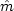is likely to be from the true mean m for a sample of length n. This difference is the variance of the sample mean and is given by, where(23) (24)
Now use the fact that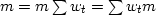:(25) (26) (27)
The step from (26) to (27) follows because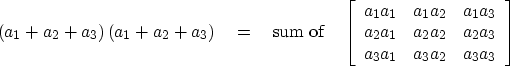(28)
The expectation symbolcan be regarded as another summation, which can be done after, as well as before, the sums on t and s, so(29)
If, since xt and xs are independent of each other, the expectation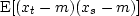will vanish. If s = t, then the expectation is the variance defined by (13). Expressing the result in terms of the Kronecker delta,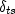(which equals unity if t=s, and vanishes otherwise) gives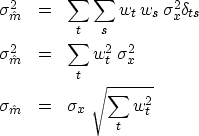(30) (31) (32)

For n weights, each of size 1/n, the standard deviation of the sample mean is(33)

This is the most important property of random numbers that is not intuitively obvious. Informally, the result (33) says this: given a sum y of terms with random polarity, whose theoretical mean is zero, then(34)
The sum y is a random variable whose standard deviation is.An experimenter who does not know the mean is zero will report that the mean of y is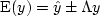,where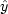is the experimental value.

If we are trying to estimate the mean of a random series that has a time-variable mean, then we face a basic dilemma. Including many numbers in the sum in order to make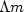small conflicts with the possibility of seeing mt change during the measurement.

The variance of the sample variance'' arises in many contexts. Suppose we want to measure the storminess of the ocean. We measure water level as a function of time and subtract the mean. The storminess is the variance about the mean. We measure the storminess in one minute and call it a sample storminess. We compare it to other minutes and other locations and we find that they are not all the same. To characterize these differences, we need the variance of the sample variance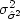.

Some of these quantities can be computed theoretically, but the computations become very cluttered and dependent on assumptions that may not be valid in practice, such as that the random variables are independently drawn and that they have a Gaussian probability function. Since we have such powerful computers, we might be better off ignoring the theory and remembering the basic principle that a function of random numbers is also a random number. We can use simulation to estimate the function's mean and variance.

Basically we are always faced with the same dilemma: if we want to have an accurate estimation of the variance, we need a large number of samples, which limits the possibility of measuring a time-varying variance.

## EXERCISES:

1. Suppose the mean of a sample of random numbers is estimated by a triangle weighting function, i.e.,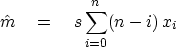Find the scale factor s so that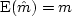.Calculate.Define a reasonable.Examine the uncertainty relation.
2. A random series xt with a possibly time-variable mean may have the mean estimated by the feedback equation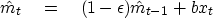a.
Expressas a function ofand not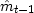.
b.
What is, the effective averaging time?

c.
Find the scale factor b so that if mt = m, thend.
Compute the random error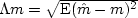.(HINT:goes to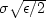as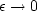.)

e.
What is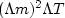in this case?Next: SPECTRAL FLUCTUATIONS Up: TIME-STATISTICAL RESOLUTION Previous: Sample mean
Stanford Exploration Project
10/21/1998